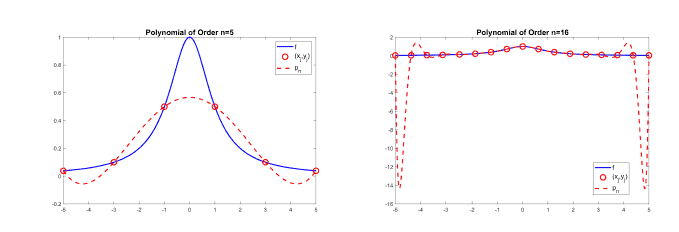## Key concepts:

• Taylor polynomials
• Taylor’s Theorem
• Polynomial interpolants
• Bernstein basis polynomials
• Bernstein polynomials
• Weierstrass Approximation Theorem

## Outline:

You should already have some appreciation for the power and importance of functions in
mathematics and mathematical applications. We have recently focused on dynamical systems
and di erential equations, which are common ways of quantitatively describing systems in
physics, etc. We used Newton’s method to generate a sequence of numbers to approximate
the solution of an equation and similarly generated a sequence of functions that converges
to the solution of a di erential equation.
The example of IVPs illustrates a more general situation where we construct mathematical
models but aren’t able to easily, or at all, express the solution as simple combinations of
polynomials and other elementary functions. In many cases, we employ numerical methods
to approximately solve our equations.
How can we digitally represent an arbitrary function of a real variable? Even on a bounded
domain [a; b] there are in nitely many points, hence, in nitely function values that need to
be de ned. Clearly, we can’t program an arbitrary function if we only have a nite amount
of computer memory.
Typically, we will instead consider a subspace of functions. For example, functions of the form
f(x) = xn are standard and come built-in with programming languages. You can construct
any polynomial by taking nite linear combinations of these monomials. Not every function
is a polynomial, of course, but is it possible to approximate any function by a polynomial?
We rst cover Taylor polynomials and polynomial interpolants, two simple but
awed strate-
gies for approximating functions. We then establish the important result that any continuous
function on [a; b] can be uniformly approximated by a sequence of polynomials. This is known
as theWeierstrass Approximation Theorem. The proof that we present makes use of a special
family of polynomials called Bernstein basis polynomials.

## Polynomial interpolants

Interpolation is about finding a function (such as a polynomial) that passes through a certain set of discrete data points. Given a (continuous? differentiable?) function $f:[a, b] \rightarrow \mathbb{R}$, we might expect a polynomial interpolation to reasonably approximate $f$ if we choose a large enough collection of data points to interpolate between.

Define $n+1$ evenly spaced points $x_{j}=a+\frac{j}{n}(b-a)$, for $j=0,1, \ldots, n$ and define $y_{j}=f\left(x_{j}\right)$ for each $j$. There is a unique polynomial $p_{n}$ of degree at most $n$ that satisfies $p_{n}\left(x_{j}\right)=y_{j}$ for $j=0,1, \ldots, n$. This $p_{n}$ is a polynomial interpolant of $f$ on the points $\left{x_{j}\right}$. See Figure 11.3

The sequence $\left(p_{n}\right)$ of polynomial interpolants obtained in this way does not always converge “nicely” to $f$. For example, for $f(x)=\frac{1}{1+x^{2}}$ on the interval $[-5,5]$, not only does the sequence $p_{n}$ not converge uniformly, but $\lim {n \rightarrow \infty}\left|f-p{n}\right|_{\infty}=\infty$. See Figure $11.4$.

Note that we chose to have the points $x_{j}$ spaced evenly apart but we don’t have to follow this convention. Any set of points can be used for interpolation, as long as the $x_{j}$ are unique (not duplicated). In the example above with $f(x)=\frac{1}{1+x^{2}}$, if you define the $n+1$ points $x_{j}$ with a different pattern or distribution over the interval $[-5,5]$, it is possible to have uniform convergence of $p_{n}$ to $f$

Unfortunately, it has been shown that no matter how you distribute $x_{j}$, there will exist a continuous function $f$ for which the sequence of interpolating polynomials does not converge uniformly to $f$Polynomial interpolation of $f(x)=\sin (2 x)$ on $[-1,1]$ with evenly spaced (in $x$ ) data points. The polynomial interpolation $p_{n}$ is almost indistinguishable from $f$ when $n=11$.

## Uniform approximations of continuous functions by polynomials

Evidently, Taylor polynomials and polynomial interpolants do not generally provide good uniform
approximations of functions. We will show that it is, in fact, always possible to uniformly approxi-
mate a continuous function on a closed interval by polynomials:

Theorem 1.

(Weierstrass Approximation Theorem). Every continuous function $f:[a, b] \rightarrow \mathbb{R}$ is the uniform limit of a sequence of polynomials $\left(p_{n}\right)$.

Weierstrass Approximation Theorem可以很容易的用三角级数的逼近得到证明，虽然狄利克雷kernel不是good kernel但是用来证明这个定理已经够用了。Polynomial interpolation of $f(x)=\frac{1}{1+x^{2}}$ on $[-5,5]$ with evenly spaced (in $x$ ) data points. Increasing $n$ gives a better match in the centre of the domain but the error in between interpolation points grows with $n$ near the endpoints of the interval.

## Bernstein basis polynomials

Bernstein basis polynomials are polynomials of the form
$$P_{k}^{n}(x)=\left(\begin{array}{l} n \ k \end{array}\right) x^{k}(1-x)^{n-k}$$
for integers $n \geq 0$ and $0 \leq k \leq n .$ The notation $\left(\begin{array}{l}n \ k\end{array}\right)$ signifies the binomial coefficient defined as $\left(\begin{array}{l}n \ k\end{array}\right)=\frac{n !}{k !(n-k) !}$
Recall the binomial formula
$$(x+y)^{n}=\sum_{k=0}^{n}\left(\begin{array}{l} n \ k \end{array}\right) x^{k} y^{n-k} .$$
Setting $y=1-x$ gives
$$1=\sum_{k=0}^{n}\left(\begin{array}{l} n \ k \end{array}\right) x^{k}(1-x)^{n-k}=\sum_{k=0}^{n} P_{k}^{n}(x) .$$
These polynomials are defined for all real numbers but we will focus on the interval $[0,1]$. Some properties of Bernstein basis polynomials:

1. $P_{k}^{n}$ is a polynomial of degree $n$.
2. $\sum_{k=0}^{n} P_{k}^{n}(x)=1$ for all $x \in[0,1]$.
3. $P_{k}^{n}(x) \geq 0$ for all $x \in[0,1]$.
4. $P_{k}^{n}(0)=0$ unless $k=0$ and $P_{k}^{n}(1)=0$ unless $k=n$.
5. $P_{k}^{n}$ attains its maximum at $x=k / n$.